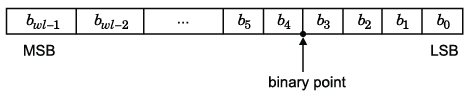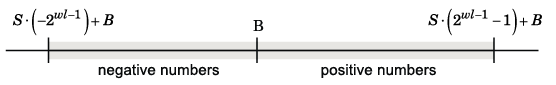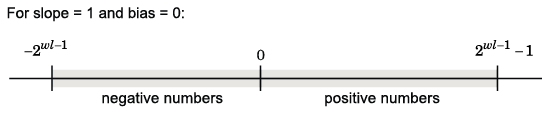## Fixed-Point Concepts and Terminology

### Fixed-Point Data Types

In digital hardware, numbers are stored in binary words. A binary word is a fixed-length sequence of bits (1s and 0s). The way hardware components or software functions interpret this sequence of 1s and 0s is defined by the data type.

Binary numbers are represented as either floating-point or fixed-point data types. In this section, we discuss many terms and concepts relating to fixed-point numbers, data types, and mathematics.

A fixed-point data type is characterized by the word length in bits, the position of the binary point, and the signedness of a number which can be signed or unsigned. Signed numbers and data types can represent both positive and negative values, whereas unsigned numbers and data types can only represent values that are greater than or equal to zero.

The position of the binary point is the means by which fixed-point values are scaled and interpreted.

For example, a binary representation of a generalized fixed-point number (either signed or unsigned) is shown below:where

• bi is the ith binary digit.

• wl is the number of bits in a binary word, also known as word length.

• bwl–1 is the location of the most significant, or highest, bit (MSB). In signed binary numbers, this bit is the sign bit which indicates whether the number is positive or negative.

• b0 is the location of the least significant, or lowest, bit (LSB). This bit in the binary word can represent the smallest value. The weight of the LSB is given by:

`$weigh{t}_{LSB}={2}^{-fractionlength}$`

where, fractionlength is the number of bits to the right of the binary point.

• Bits to the left of the binary point are integer bits and/or sign bits, and bits to the right of the binary point are fractional bits. Number of bits to the left of the binary point is known as the integer length. The binary point in this example is shown four places to the left of the LSB. Therefore, the number is said to have four fractional bits, or a fraction length of four.

Fixed-point data types can be either signed or unsigned.

Signed binary fixed-point numbers are typically represented in one of three ways:

• Sign/magnitude –– Representation of signed fixed-point or floating-point numbers. In the sign/magnitude representation, one bit of a binary word is always the dedicated sign bit, while the remaining bits of the word encode the magnitude of the number. Negation using sign/magnitude representation consists of flipping the sign bit from 0 (positive) to 1 (negative), or from 1 to 0.

• One's complement

• Two's complement –– Two's complement is the most common representation of signed fixed-point numbers. See Two's Complement for more information.

Unsigned fixed-point numbers can only represent numbers greater than or equal to zero.

### Scaling

In [Slope Bias] representation, fixed-point numbers can be encoded according to the scheme

`$real\text{-}worldvalue=\left(slope×integer\right)+bias$`

where the slope can be expressed as

The term slope adjustment is sometimes used as a synonym for fractional slope.

In the trivial case, slope = 1 and bias = 0. Scaling is always trivial for pure integers, such as int8, and also for the true floating-point types single and double.

The integer is sometimes called the stored integer. This is the raw binary number, in which the binary point assumed to be at the far right of the word. In System Toolboxes, the negative of the exponent is often referred to as the fraction length.

The slope and bias together represent the scaling of the fixed-point number. In a number with zero bias, only the slope affects the scaling. A fixed-point number that is only scaled by binary point position is equivalent to a number in the Fixed-Point Designer™ [Slope Bias] representation that has a bias equal to zero and a slope adjustment equal to one. This is referred to as binary point-only scaling or power-of-two scaling:

or

In System Toolbox software, you can define a fixed-point data type and scaling for the output or the parameters of many blocks by specifying the word length and fraction length of the quantity. The word length and fraction length define the whole of the data type and scaling information for binary-point only signals.

All System Toolbox blocks that support fixed-point data types support signals with binary-point only scaling. Many fixed-point blocks that do not perform arithmetic operations but merely rearrange data, such as Delay and Matrix Transpose, also support signals with [Slope Bias] scaling.

### Precision and Range

You must pay attention to the precision and range of the fixed-point data types and scalings you choose for the blocks in your simulations, in order to know whether rounding methods will be invoked or if overflows will occur.

#### Range

The range is the span of numbers that a fixed-point data type and scaling can represent. The range of representable numbers for a two's complement fixed-point number of word length wl, scaling S, and bias B is illustrated below:For both signed and unsigned fixed-point numbers of any data type, the number of different bit patterns is 2wl.

For example, in two's complement, negative numbers must be represented as well as zero, so the maximum value is 2wl–1. Because there is only one representation for zero, there are an unequal number of positive and negative numbers. This means there is a representation for -2wl–1 but not for 2wl–1:The full range is the broadest range for a data type. For floating-point types, the full range is –∞ to ∞. For integer types, the full range is the range from the smallest to largest integer value (finite) the type can represent. For example, from -128 to 127 for a signed 8-bit integer.

Overflow Handling.  Because a fixed-point data type represents numbers within a finite range, overflows can occur if the result of an operation is larger or smaller than the numbers in that range.

System Toolbox software does not allow you to add guard bits to a data type on-the-fly in order to avoid overflows. Guard bits are extra bits in either a hardware register or software simulation that are added to the high end of a binary word to ensure that no information is lost in case of overflow. Any guard bits must be allocated upon model initialization. However, the software does allow you to either saturate or wrap overflows. Saturation represents positive overflows as the largest positive number in the range being used, and negative overflows as the largest negative number in the range being used. Wrapping uses modulo arithmetic to cast an overflow back into the representable range of the data type. See Modulo Arithmetic for more information.

#### Precision

The precision of a fixed-point number is the difference between successive values representable by its data type and scaling, which is equal to the value of its least significant bit. The value of the least significant bit, and therefore the precision of the number, is determined by the number of fractional bits. A fixed-point value can be represented to within half of the precision of its data type and scaling. The term resolution is sometimes used as a synonym for this definition.

For example, a fixed-point representation with four bits to the right of the binary point has a precision of 2-4 or 0.0625, which is the value of its least significant bit. Any number within the range of this data type and scaling can be represented to within (2-4)/2 or 0.03125, which is half the precision. This is an example of representing a number with finite precision.

Rounding Modes.  When you represent numbers with finite precision, not every number in the available range can be represented exactly. If a number cannot be represented exactly by the specified data type and scaling, it is rounded to a representable number. Although precision is always lost in the rounding operation, the cost of the operation and the amount of bias that is introduced depends on the rounding mode itself. To provide you with greater flexibility in the trade-off between cost and bias, DSP System Toolbox™ software currently supports the following rounding modes:

• `Ceiling` rounds the result of a calculation to the closest representable number in the direction of positive infinity.

• `Convergent` rounds the result of a calculation to the closest representable number. In the case of a tie, `Convergent` rounds to the nearest even number. This is the least biased rounding mode provided by the toolbox.

• `Floor`, which is equivalent to truncation, rounds the result of a calculation to the closest representable number in the direction of negative infinity. The truncation operation results in dropping of one or more least significant bits from a number.

• `Nearest` rounds the result of a calculation to the closest representable number. In the case of a tie, `Nearest` rounds to the closest representable number in the direction of positive infinity.

• `Round` rounds the result of a calculation to the closest representable number. In the case of a tie, `Round` rounds positive numbers to the closest representable number in the direction of positive infinity, and rounds negative numbers to the closest representable number in the direction of negative infinity.

• `Simplest` rounds the result of a calculation using the rounding mode (`Floor` or `Zero`) that adds the least amount of extra rounding code to your generated code. For more information, see Rounding Mode: Simplest (Fixed-Point Designer).

• `Zero` rounds the result of a calculation to the closest representable number in the direction of zero.